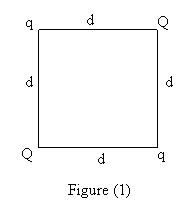KFUPM-Physics102-032
Dr.Aljalal
Sec07
Sec08
Sec09

Recitation ch 22

Question 1
Two point charges q1 and q2 lie along the x-axis.
q1 = + 16.0 micro-Coulombs is at x = 2.00 m and
q2 = + 9.00 micro-Coulombs is at the origin.
Where must a negative charge q3 be placed on
the x-axis such that the net electrostatic force
on it is zero ?

a   x = + 1.14 m
b   x = - 1.14 m
c   x = + 0.857 m
d   x = - 0.857 m
e   x = + 2.86 m
==============================================
Question 2
As in figure (1), a charge Q is fixed at each of two opposite
corners of a square. A charge q is fixed at each of the other
two corners. If the resultant electrical force on Q is zero,
then Q and q are related as:`a  Q = - 2 Sqrt(2) q**2
b  Q = q
c  Q = - 2 Sqrt(2) q
d  Q = q**2
e  Q = - 4 q
==============================================
Question 3
Two fixed particles, of charges q1 = + 1.0*10**(-6) C and q2=
- 9.0*10**(-6) C, are 10 cm apart. How far from each should
a third charge be located so that no net electrostatic force
acts on it?

a  1 cm from q1 and 11 cm from q2.
b  5 cm from q1 and 15 cm from q2.
c  3 cm from q1 and 7 cm from q2.
d  1 cm from q1 and 9 cm from q2.
e  1.1 cm from q1 and 11.1 cm form q2.
==============================================
Question 4
Consider two identical conductor spheres, A and B.
Initially, sphere A has a charge of -80 Q and Sphere B
has a charge of +20 Q. If the spheres touched and then
are separated by a distance of 0.3 m, what is the
resultant force between them? [Take Q = 5.7*10**(-8) C]

a   0.2 N.
b   0.3 N.
c   0.4 N.
d   0.6 N.
e   0.9 N.
==============================================
Question 5
Charges q1 and q2 are on the x-axis. q1 is at x = a
and q2 is at x = 2a. The net force on a third charge
at the origin is zero.
Which of the following is TRUE ?

a   q2 = -4*q1
b   q2 = 2*q1
c   q2 = -q1
d   q2 = 4*q1
e   q2 = -2*q1
==============================================